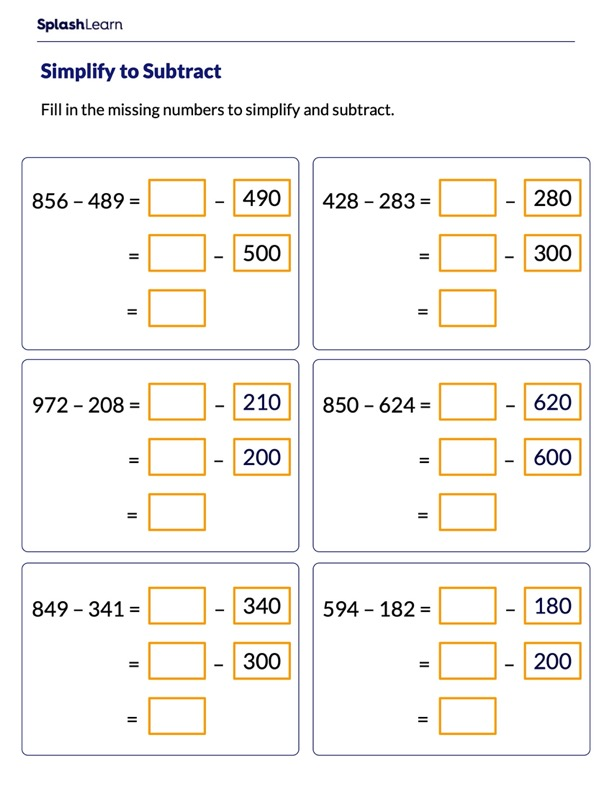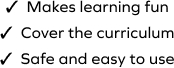# Subtract by Making Multiples of 100

SplashLearn - The complete PreK - Grade 5 Math & ELA Learning Program Built for Your Child
Home > Subtract by Making Multiples of 100Struggles with subtraction strategies can easily be overcome if students practice the concept in a fun and engaging way! The worksheet gives students plenty of opportunities to work with problems based on subtraction. They are encouraged to apply their mathematical knowledge to find the missing numbers in math sentences. Students will apply "make a multiple of 100 to subtract" strategy to solve the given problems.